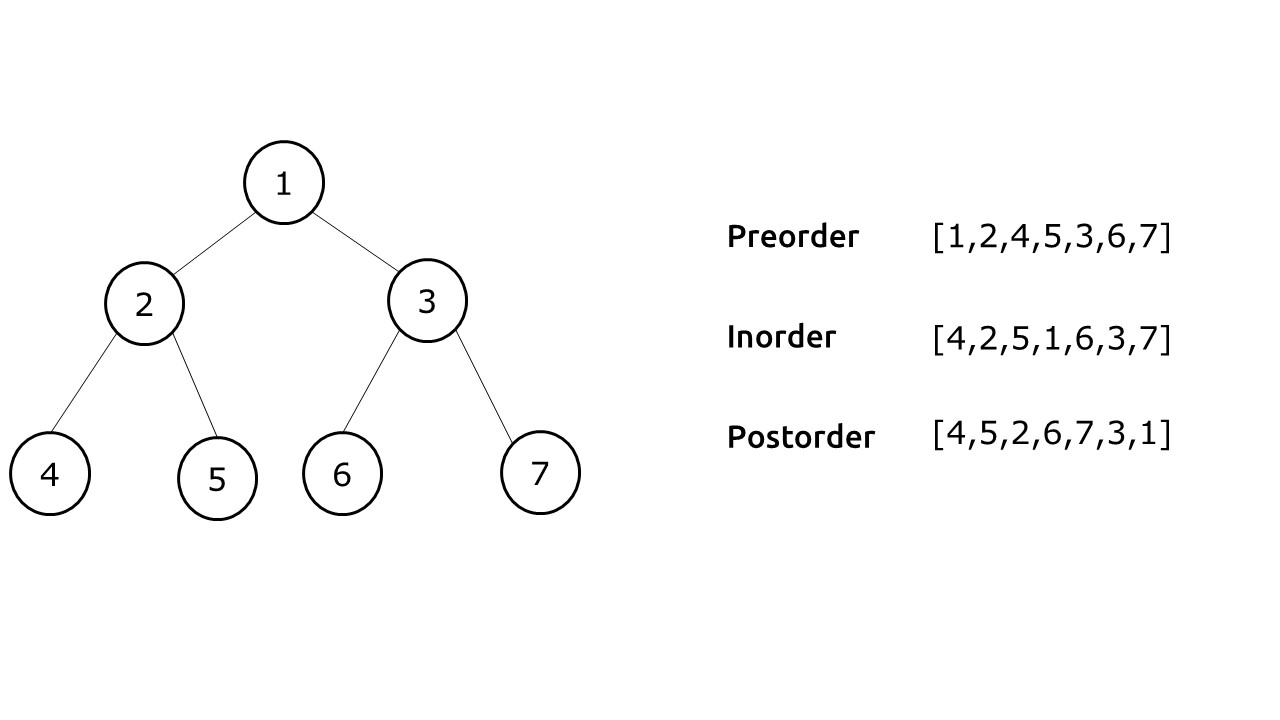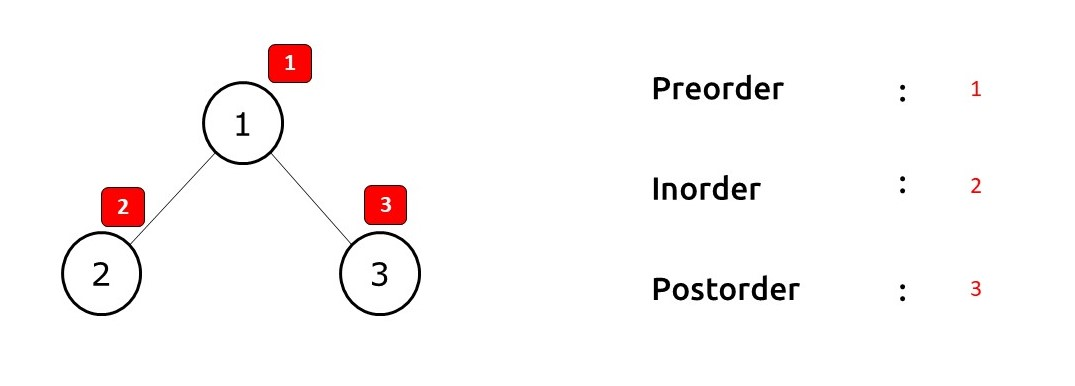# Preorder Inorder Postorder Traversals in One Traversal

Problem Statement: Preorder Inorder Postorder Traversals in One Traversal. Write a program to print Preorder, Inorder, and Postorder traversal of the tree in a single traversal.

```Example:```

Problem Description:

We need to write a function allTraversals() which returns us three lists of a preorder, inorder and postorder traversals of the tree at the same time. We are not allowed to write separate codes for each traversal. We want all traversals in a single piece of code, in a single instance.

Disclaimer: Don’t jump directly to the solution, try it out yourself first.

Pre-req: Traversals Video, Stack Data Structure

### Solution :

Intuition: If we study the preorder, inorder and postorder traversals, we will observe a pattern. To understand this pattern, we take a simple example:The number inside the red boxes is the visit when we print the node. In preorder traversal, we print a node at the first visit itself. Whereas, in inorder traversal at the first visit to a node, we simply traverse to the left child. It is only when we return from the left child and visit that node the second time, that we print it. Similarly, in postorder traversal, we print a node in its third visit after visiting both its children.

Approach:

The algorithm steps can be described as follows:

We take a stack data structure and push a pair<val, num> to it. Here Val is the value of the root node and num the visit to the node. Initially, the num is 1 as we are visiting the root node for the first time. We also create three separate lists for preorder, inorder and postorder traversals. Then we set an iterative loop to run till the time our stack is non-empty.

In every iteration, we pop the top of the stack (say, T). Then we check the second value(num) of T. Three cases can arise:

• Case 1 : When num==1

This means that we are visiting the node for the very first time, therefore we push the node value to our preorder list. Then we push the same node with num=2(for Case 2). After this, we want to visit the left child. Therefore we make a new pair Y(<val, num>) and push it to the stack (if there exists a left child). The val of Y is equal to the left child’s node value and num is equal to 1.

• Case 2 : When num==2

This means that we are visiting the node for the second time, therefore on our second visit to the node, we push the node value to our inorder list. Then we push the same node with num=3( for Case 3). After this, we want to visit the right child. Therefore as in the first case, we check if there exists a right child or not. If there is, we push the right child and num value=1 as a pair to our stack.

• Case 3 When num==3

This means that we are visiting the node for the third time. Therefore we will push that node’s value to our postorder list. Next, we simply want to return to the parent so we will not push anything else to the stack.

Dry Run: In case you want to watch the dry run for this approach, please watch the video attached below.

Code:

## C++ Code

``````#include <bits/stdc++.h>

using namespace std;

struct node {
int data;
struct node * left, * right;
};

void allTraversal(node * root, vector < int > & pre, vector < int > & in , vector < int > & post) {
stack < pair < node * , int >> st;
st.push({
root,
1
});
if (root == NULL) return;

while (!st.empty()) {
auto it = st.top();
st.pop();

// this is part of pre
// increment 1 to 2
// push the left side of the tree
if (it.second == 1) {
pre.push_back(it.first -> data);
it.second++;
st.push(it);

if (it.first -> left != NULL) {
st.push({
it.first -> left,
1
});
}
}

// this is a part of in
// increment 2 to 3
// push right
else if (it.second == 2) {
in .push_back(it.first -> data);
it.second++;
st.push(it);

if (it.first -> right != NULL) {
st.push({
it.first -> right,
1
});
}
}
// don't push it back again
else {
post.push_back(it.first -> data);
}
}
}

struct node * newNode(int data) {
struct node * node = (struct node * ) malloc(sizeof(struct node));
node -> data = data;
node -> left = NULL;
node -> right = NULL;

return (node);
}

int main() {

struct node * root = newNode(1);
root -> left = newNode(2);
root -> left -> left = newNode(4);
root -> left -> right = newNode(5);
root -> right = newNode(3);
root -> right -> left = newNode(6);
root -> right -> right = newNode(7);

vector < int > pre, in , post;
allTraversal(root, pre, in , post);

cout << "The preorder Traversal is : ";
for (auto nodeVal: pre) {
cout << nodeVal << " ";
}
cout << endl;
cout << "The inorder Traversal is : ";
for (auto nodeVal: in ) {
cout << nodeVal << " ";
}
cout << endl;
cout << "The postorder Traversal is : ";
for (auto nodeVal: post) {
cout << nodeVal << " ";
}
cout << endl;

return 0;
}``````

Output:

The preorder Traversal is: 1 2 4 5 3 6 7
The inorder Traversal is: 4 2 5 1 6 3 7
The postorder Traversal is: 4 5 2 6 7 3 1

Time Complexity: O(N)

Reason: We are visiting every node thrice therefore time complexity will be O(3*N), which can be assumed as linear time complexity.

Space Complexity: O(N)

Reason: We are using three lists and a stack to store the nodes. The time complexity will be about O(4*N), which can be assumed as linear time complexity.

## Java Code

``````import java.util.*;
class TreeNode {
int val;
TreeNode left;
TreeNode right;
TreeNode() {}
TreeNode(int val) {
this.val = val;
}
}
class Pair {
TreeNode node;
int num;
Pair(TreeNode _node, int _num) {
num = _num;
node = _node;
}
}
public class TUF {
public static void allTraversal(TreeNode root, List < Integer > pre, List < Integer > in , List < Integer > post) {
Stack < Pair > st = new Stack < Pair > ();
st.push(new Pair(root, 1));

if (root == null) return;

while (!st.isEmpty()) {
Pair it = st.pop();

// this is part of pre
// increment 1 to 2
// push the left side of the tree
if (it.num == 1) {
it.num++;
st.push(it);

if (it.node.left != null) {
st.push(new Pair(it.node.left, 1));
}
}

// this is a part of in
// increment 2 to 3
// push right
else if (it.num == 2) { in .add(it.node.val);
it.num++;
st.push(it);

if (it.node.right != null) {
st.push(new Pair(it.node.right, 1));
}
}
// don't push it back again
else {
}
}

}
public static void main(String args[]) {

TreeNode root = new TreeNode(1);
root.left = new TreeNode(2);
root.left.left = new TreeNode(4);
root.left.right = new TreeNode(5);
root.right = new TreeNode(3);
root.right.left = new TreeNode(6);
root.right.right = new TreeNode(7);

List < Integer > pre = new ArrayList < > ();
List < Integer > in = new ArrayList < > ();
List < Integer > post = new ArrayList < > ();
allTraversal(root, pre, in , post);

System.out.println("The preorder Traversal is : ");
for (int nodeVal: pre) {
System.out.print(nodeVal + " ");
}
System.out.println();
System.out.println("The inorder Traversal is : ");
for (int nodeVal: in ) {
System.out.print(nodeVal + " ");
}
System.out.println();
System.out.println("The postorder Traversal is : ");
for (int nodeVal: post) {
System.out.print(nodeVal + " ");
}
System.out.println();
}
}``````

Output:

The preorder Traversal is :
1 2 4 5 3 6 7
The inorder Traversal is :
4 2 5 1 6 3 7
The postorder Traversal is :
4 5 2 6 7 3 1

Time Complexity: O(N)

Reason: We are visiting every node thrice therefore time complexity will be O(3*N), which can be assumed as linear time complexity.

Space Complexity: O(N)

Reason: We are using three lists and a stack to store the nodes. The time complexity will be about O(4*N), which can be assumed as linear time complexity.